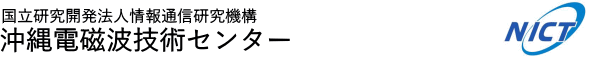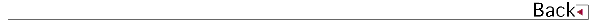# 2014\$BG/EY(B (2014.04\$B!A(B2015.03)

## \$B8&5fO@J8(B

• \$B8eF#(B \$BCi9-(B, \$B5WJ]2,(B \$B=S9((B, \$B1+C+(B \$B=c(B, \$B;~9oHf3SMQ%=%U%H%&%'%"(BGPS\$BpJsDL?.3X2q(B \$B!!O@J8;o(BB.
• \$B@nH*(B \$BBsLp(B, \$B4d0f(B \$B9(FA(B, \$B@%8E(B \$B90(B, \$B>.;J(B \$BDw65(B, \$B@FF#(B \$BOBM:(B, \$B@P0f(B \$B>;7{(B, \$B?eC+(B \$B9LJ?(B, Cloud-Resolving 4D-Var Assimilation of Doppler Wind Lidar Data on a Meso-gamma Scale Convective System, Monthly Weather Review.

## \$B<}O?O@J8(B

• \$B8eF#(B \$BCi9-(B, \$B5WJ]2,(B \$B=S9((B, \$B1+C+(B \$B=c(B, \$BJ|Aw1R@1\$rCg2p\$H\$7\$?F|K\I8=`;~G[?.J}K!\$N8!F\$#2(B, \$BBh(B58\$B2s1'Ch2J3X5;=QO"9g9V1i2q(B.

## \$B0lHL8}F,H/I=(B

• \$B4d0f(B \$B9(FA(B, \$B@DLZ(B \$B@?(B, \$BCf@n(B \$B>!9-(B, \$B%I%C%W%i!<%i%\$%@!<\$K\$h\$k1+E)Mn2]3X2q(B2014\$BG/EY=)5(Bg2q(B.
• \$B4d0f(B \$B9(FA(B, \$B@P0f(B \$B>;7{(B, \$B?eC+(B \$B9LJ?(B, Baron Philippe, \$BCf@n(B \$B>!9-(B, \$B@nB<(B \$B@?<#(B, \$BMn9g(B \$B7<(B, \$B0B0f(B \$B85><(B, \$B1:DM(B \$B@6Jv(B, \$BAjGO(B \$B0l5A(B, \$BBg@P(B \$BE/(B, \$B;38}(B \$B90@?(B, \$BCfKL(B \$B1Q0l(B, \$B%I%C%W%i!<%i%\$%@!<\$K\$h\$j4QB,\$5\$l\$?O;9C\$*\$m\$7\$N9=B\$(B, \$BF|K\5\$>]3X2q(B2014\$BG/EY=U5(Bg2q(B.
• \$B?yC+(B \$BLPIW(B, \$B4d0f(B \$B9(FA(B, \$B?9K\(B \$B>
• \$B?yC+(B \$BLPIW(B, \$B1+C+(B \$B=c(B, 60GHz\$BBSEAAw\$K\$h\$k5?;w;(2;?.9f\$rMQ\$\$\$?9b@:EYF14|?.9f6!5kAuCV\$N3+H/(B, \$BEE;R>pJsDL?.3X2q(B.
• \$B?yC+(B \$BLPIW(B, \$B?9K\(B \$B>
• \$B?yC+(B \$BLPIW(B, \$B1+C+(B \$B=c(B, \$B4d0f(B \$B9(FA(B, \$BJ,;67?3\$MN%l!<%@3+H/\$K\$`\$1\$F\$N9b@:EYF14|?.9f6!5k%7%9%F%`3+H/(B, \$B6e=#Bg3X1~MQNO3X8&5f=j(B \$B6&F18&5f=82q(B.
• \$B8eF#(B \$BCi9-(B, \$B1+C+(B \$B=c(B, \$B2VEZ(B \$B90(B, \$B@nB<(B \$B@?<#(B, \$BAjED(B \$B@/B'(B, \$B6(D4@)8f7?%l!<%@F14|It\$N;n:n(B, \$BEE;R>pJsDL?.3X2q(B2015\$BG/Am9gBg2q(B.
• \$B8eF#(B \$BCi9-(B, \$B1+C+(B \$B=c(B, \$BAjED(B \$B@/B'(B, \$B2VEZ(B \$B90(B, \$B@nB<(B \$B@?<#(B, \$B:4F#(B \$B?82p(B, \$B;T@n(B \$BN40l(B, \$B<~GH?t\$NM-8zMxMQ\$rL\E*\$H\$7\$?6(D4@)8f7?5\$>]%l!<%@%7%9%F%`\$N8!F\$(B \$B\$=\$N#5(B \$B%l!<%@F14|5;=Q\$NZ]3X2q(B2014\$BG/EY=U5(Bg2q(B.
• \$B@DLZ(B \$B@?(B, \$B4d0f(B \$B9(FA(B, \$BCf@n(B \$B>!9-(B, \$B?eC+(B \$B9LJ?(B, PANDA\$B%I%C%W%i!<%i%\$%@!<\$NIwB,Dj@:EY8!>Z(B -GPS\$B%>%s%G\$*\$h\$SD62;GHIwB.7WB,Dj\$H\$NHf3S(B-, \$B1~MQJ*M}3X2q(B.
• \$B@DLZ(B \$B@?(B, \$B4d0f(B \$B9(FA(B, \$BCf@n(B \$B>!9-(B, \$B@P0f(B \$B>;7{(B, \$B?eC+(B \$B9LJ?(B, \$B%U%'!<%:%I%"%l%\$5\$>]%l!<%@!&%I%C%W%i!<%i%\$%@!
• \$B@DLZ(B \$B@?(B, \$B4d0f(B \$B9(FA(B, \$BCf@n(B \$B>!9-(B, \$B?eC+(B \$B9LJ?(B, PANDA\$B%I%C%W%i!<%i%\$%@!<\$NIwB,Dj@:EY8!>Z(B,PANDA\$B%I%C%W%i!<%i%\$%@!<\$NIwB,Dj@:EY8!>Z(B.
• \$B@DLZ(B \$B@?(B, \$B4d0f(B \$B9(FA(B, \$BCf@n(B \$B>!9-(B, \$B?eC+(B \$B9LJ?(B, \$B%U%'!<%:%I%"%l%\$5\$>]%l!<%@!&%I%C%W%i!<%i%\$%@!
• \$B;3ED(B \$B9-9,(B, \$B4d0f(B \$B9(FA(B, 2010\$BG/1bH~9k1+\$N4|4VCf\$KKL\$XEAGE\$9\$k9_?e%7%9%F%`(B, \$BF|K\5\$>]3X2q(B2014\$BG/EY=U5(Bg2q(B.
• \$B?9K\(B \$B>Z(B, \$BF|K\3\$MN3X2q(B.
• \$B?9K\!!>R2p(B, Ocean Radar Conference for Asia-Pacific.
• \$B:4F#(B \$B?82p(B, \$B0kED(B \$BAm;R(B, \$B4d0f(B \$B9(FA(B, \$BCf@n(B \$B>!9-(B, \$B2VEZ(B \$B90(B, \$B9b66(B \$BD*9((B, \$BLZED(B \$BCR;K(B, \$B?eC+(B \$BJ8I'(B, \$BJ?Ln(B \$BM54p(B, \$BEhB<(B \$B=E<#(B, \$B5mHx(B \$BCNM:(B, \$B#2Bf\$N%U%'!<%:%I%"%l%\$5\$>]%l!<%@!<\$K\$h\$k#3l\$N4QB,(B, \$BL>8E20Bg3XCO5e?e=[4D8&5f%;%s%?!<8&5f=82q(B.
• \$BCf@n(B \$B>!9-(B, \$B2VEZ(B \$B90(B, \$B4d0f(B \$B9(FA(B, \$B@nB<(B \$B@?<#(B, \$B9b66(B \$BD*9((B, \$B0f8}(B \$B=SIW(B, NICT \$B\$K\$*\$1\$k(B GPM/DPR \$B\$NCO>e8!>Z7W2h(B, \$BF|K\5\$>]3X2q(B.
• \$B:4F#(B \$B?82p(B, \$B0kED(B \$BAm;R(B, \$B4d0f(B \$B9(FA(B, \$BCf@n(B \$B>!9-(B, \$B2VEZ(B \$B90(B, \$B9b66(B \$BD*9((B, \$BLZED(B \$BCR;K(B, \$B?eC+(B \$BJ8I'(B, \$BJ?Ln(B \$BM54p(B \$BEhB<(B \$B=E<#(B \$B5mHx(B \$BCNM:(B, \$B#2Bf\$N%U%'!<%:%I%"%l%\$5\$>]%l!<%@!<\$K\$h\$kBPN.1@\$N#3l\$N4QB,(B, \$BF|K\5\$>]3X2q(B2014\$BG/EY=)5(Bg2q(B.
• \$B8EK\(B \$B=_0l(B, \$B:4F#(B \$B?82p(B, \$B4d0f(B \$B9(FA(B, \$B5WJ]ED(B \$BBs;V(B, \$BEl(B \$BK.><(B, \$B668}(B \$B9@G7(B, PANDA\$B\$K\$h\$k%l!<%@!]3X2q(B2014\$BG/EY=)5(Bg2q(B.
• \$BBl@n(B \$BE/B@O:(B, \$B?9K\(B \$B>]\$K4X\$9\$k6&F18&5f(B, \$BL>8E20Bg3XCO5e?e=[4D8&5f%;%s%?!<(B2015\$B8&5f=82q(B.
• \$B;T@n(B \$BN40l(B, Hobiger Thomas, \$B8eF#(B \$BCi9-(B, \$B1+C+(B \$B=c(B, \$B5\Fb(B \$B7k2V(B, \$B@.ED(B \$B=(2A(B, AOGS2014.
• \$B9b66(B \$BD*9((B, \$B0kED(B \$BAm;R(B, \$B:4F#(B \$B?82p(B, \$B4d0f(B \$B9(FA(B, \$B@DLZ(B \$B@?(B, \$BCf@n(B \$B>!9-(B, \$B2VEZ(B \$B90(B, \$B%U%'!<%:%I%"%l%\$5\$>]%l!<%@\$K\$h\$k9_?e1@\$N#3]3X2q!!(B2014\$BG/=)5(Bg2q(B.
• \$B1J>B(B \$B85(B, \$BBl@n(B \$BE/B@O:(B, \$B>.?K(B \$BE}(B, \$B?9K\(B \$B>

## \$B5(Js(B

• \$BCf@n(B \$B;K4](B, \$B9b66(B \$BLw9((B, \$BED^<(B \$BNI(B, \$B1+C+(B \$B=c(B, \$BCfB<(B \$B??HA(B, \$BEZ20(B \$BLP(B, \$BIM(B \$B??0l(B, 5-2 \$B9b@:EY;~9oHf3SAuCV\$rMQ\$\$\$?CO>e(B-\$BCO>e4V;~9oHf3SpJsDL?.8&5f5!9=8&5fJs9p(BVol.60 No.1 2014.
• \$B9b66(B \$BLw9((B, \$BT"?9(B \$BM5@8(B, \$BCf@n(B \$B;K4](B, \$BED^<(B \$BNI(B, \$B1+C+(B \$B=c(B, \$BEZ20(B \$BLP(B, \$BIM(B \$B??0l(B,5-3 \$B9b@:EY;~9oHf3SAuCV\$rMQ\$\$\$?9b@:EYB,5wpJsDL?.8&5f5!9=8&5fJs9p(BVol.60 No.1 2014.

## 1990\$B!A(B1991\$B!d(B1992\$B!d(B1993\$B!d(B1994\$B!d(B1995\$B!d(B1996\$B!d(B1997\$B!d(B1998\$B!d(B 1999\$B!d(B2000\$B!d(B2001\$B!d(B2002\$B!d(B2003\$B!d(B2004\$B!d(B2005\$B!d(B2006\$B!d(B2007\$B!d(B 2008\$B!d(B2009\$B!d(B2010\$B!d(B2011\$B!d(B2012\$B!d(B2013\$B!d(B2014\$B!d(B2015\$B!d(B2016\$B!d(B2017